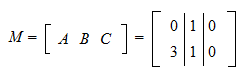StatLect

# Block matrix

A block matrix (or partitioned matrix) is a matrix that is subdivided into blocks that are themselves matrices. The subdivision is performed by cutting the matrix one or more times, vertically and/or horizontally.## Blocks

Given a matrix, a submatrix (or block) ofis a matrix that is obtained fromby deleting some of its rows and/or columns.

Example Define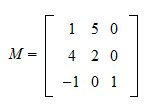Then, by deleting the second row and the third column of, we obtain the submatrixBy deleting the first column of, we obtain the submatrixRow and column vectors, despite being special matrices that have a single column or row respectively, can be used to form blocks.

Example Consider the column vectorThen, by deleting its second row, we get the blockExample Letbe the row vector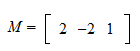Then, after striking out its third column, we are left with the submatrixIf we instead delete the first and second column of, we get## Horizontal and vertical cuts

As we said in the introduction, a block matrix is the result of performing some vertical and horizontal cuts on a matrix so as to subdivide it into blocks.

Example Define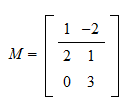where an horizontal cut has been performed between the first and the second row. Then, we can writeor simplywhereThus, the partitioned matrixis made up of the two blocksand.

Example Take the block matrixin the previous example and perform another cut, vertically, between the first and the second column. Then,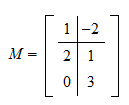Thus,where the four submatrices areWe have seen how to obtain a partitioned matrix by cutting it into blocks. Another way to obtain a partitioned matrix is to first specify the blocks and then adjoin them so as to obtain a larger matrix.

Example DefineThen, we can adjoin the four blocks to create the block matrix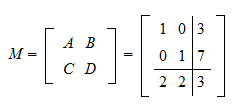As the cuts between rows and columns cannot be staggered, we need to follow these rules:

• if two or more matrices are adjoined on a row, then they must have the same number of rows;

• if two or more matrices are adjoined on a column, then they must have the same number of columns.

Example Consider the following matrix with six blocks: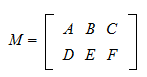Then, for instance,,andmust have the same number of rows andandmust have the same number of columns.

When matrices are adjoined on a row, we say that they are adjoined horizontally. When they are adjoined on a column, we say that they are adjoined vertically.

Example In the previous example,andare adjoined horizontally, whileandare adjoined vertically.

## Solved exercises

Below you can find some exercises with explained solutions.

### Exercise 1

Explicitly write out the blocks that result from performing 1) a horizontal cut between the first and second row, and 2) a vertical cut between the second and third column of the matrix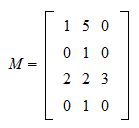Solution

After performing the cuts, the matrix can be written aswhere### Exercise 2

Find what partitioned matrix is obtained by horizontally adjoining the blocks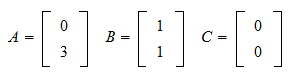Solution

The partitioned matrix is